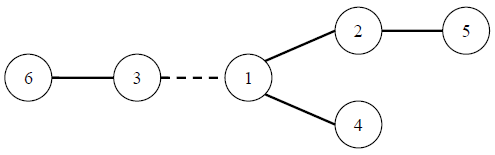## Day 2, Problem 1 - Road Construction

On planet W, there exists n countries. To promote the economic growth of each country, the kings of the countries have decided to construct bidirectional roads to ensure that all countries are connected. However, since they're all incredibly stingy, they wish to construct exactly n − 1 roads.

Constructing each road will require a cost. This cost is equal to the length of the road multiplied by the absolute difference of the number of countries on each side of the road. For example, the road represented by a dashed line in the figure below has, respectively, 2 and 4 countries on each of its sides. If this road has a length of 1, then the cost will be 1×|2 − 4| = 2. The circled numbers represent the numbers of the countries.Since both the number of countries and the number of ways to construct the roads are extremely large, as well the construction costs for each way is hard to calculate by humans, the kings have decided to hire a person to design a software to do this. This piece of software should be able calculate the total cost of constructing all the roads, given a way to construct them. Please help the kings to write such a program.

### Input Format

The first line contains an integer n, representing the number countries on planet W. Countries are numbered from 1 to n.
For the following n − 1 lines, each line will describe the construction of a single road. The i-th of these lines will contain three integers ai, bi, and ci, indicating that the i-th bidirectional road connects countries ai and bi, and has a length of ci.

### Output Format

Output a single integer, the total cost of constructing all the roads.

```6
1 2 1
1 3 1
1 4 2
6 3 1
5 2 1
```

```20
```

### Constraints

The attributes of all the test cases are outlined below.

Test CaseSize of n
(Note the =, not ≤)
Constraints
1n = 21 ≤ ai, bin
0 ≤ ci ≤ 106
2n = 10
3n = 100
4n = 200
5n = 500
6n = 600
7n = 800
8n = 1000
9n = 10,000
10n = 20,000
11n = 50,000
12n = 60,000
13n = 80,000
14n = 100,000
15n = 600,000
16n = 700,000
17n = 800,000
18n = 900,000
19n = 1,000,000
20n = 1,000,000

Point Value: 15 (partial)
Time Limit: 2.00s
Memory Limit: 256M